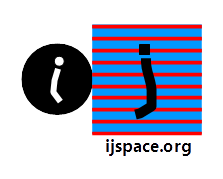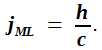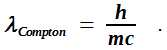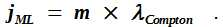A Timeless Constant 9th May 2019 HOME "An essential feature of measurement is the difference between the “determination” of an object by individual specification and the determination of the same object by some conceptual means." - Herman Weyl, Space Time Matter   "One could also ask a slightly different question. What are the principles that govern the interaction of a microscopic system with a classical system? One would like to know whether it is possible to define classical physical systems that cannot interact with quantum systems." - Rathindra Nath Sen, Causality, Measurement Theory and the Differentiable Structure of Space-Time. "Is colour metabolic efficient or coding efficient?" - James V Stone, Principles of Neural Information Theory.   "And so these men of Indostan Disputed loud and long, Each in his own opinion Exceeding stiff and strong, Tho' each was partly in the right, And all were in the wrong." - Elephant and the blind men, a childhood story. ..And it took a Witten to put the M-elephant together. In j-space the perception of space and time, are based on the measurements made by an observer, and therefore they are specific to the observer.  Different observers, unless they are following Lorentz Invariance condition, may have measurements which do not agree with each other.  Hence they may view universe differently.  For example an observer named Taku may not be able to measure certain features of the universe, features which can be measured by Baku, another observer with higher measurement capacity.    Further the existence of time is required, before the measurement of space itself can be thought of.  This requirement is crucial as the conventional space can not exist without the existence of an observer to make measurements.  For a give topology or equivalently a given path, each observer1 comes with its own time-axis based on the observer's measurement capacity.  Each observer is measuring the infinite source or the exact source.  The observers obeying the Lorentz invariance, make measurements along a congruent time-axis.     In the previous blog, we highlighted this problem of time itself not being a universal entity.  You see, each measurement is supposed to be a zero-entropy measurement, and hence no time-axis2.  But entropy inherent in the system, a system represented by the car named Bubble Aku is travelling in, does not allow a zero-entropy measurement, and subsequently the time-axis starts stretching out towards infinity.     Our objectives or at least one of them, is to come up the description of a topological space which is being measured by Aku, and this topological space must be time independent.  Can we figure out based on Aku's measurements which are assisted by precision observers 133Cs and HeNe, a constant which is time independent?  The universal constants available to Aku are h, c, and G.  We can also include kB, but it does not represents relativistic limits.3     Let us consider for example h and c first and perform dimensional analysis.  The dimensions of h and c are [M L2 T-1]  and [LT-1].  So we need to cancel out the time-specific term, which is T.  Let us take a simple ratio4 of h and c and define:jML represents a constant in discrete-measurement space or j-space.  The subscripts ML remind us that the constant jML, has the dimensions of mass multiplied by length, and it is independent of time.     Next question to ask, is what does jML really represent?  Turns out that we do not have to go far.  We are already familiar with Compton wavelength, a property associated with a particle in its relativistic limits.  Compton wavelength is given as:In this formula, h is the Planck constant, m is the mass associated with a particle or a structure , and c is the velocity of light.  An interesting topic of discussion would be the nature of the mass m, whether it is inertial or gravitational.  For the time being, we assume that the inertial and the gravitational mass are equal in topological space.  Therefore we can write jML in terms of Compton wavelength λCompton as:The dimensions of jML remain mass × length and hence timeless.  At this point it will be prudent to discuss the significance of Compton wavelength.  The physics behind Compton scattering can be found in the Wikipedia article here.  We want to understand how λCompton provides a better understanding of structures with different information contents when they are measured in j-space, and if jML can truly be considered a topological constant in a discrete measurement space or j-space.     In terms of photon-scattering, λCompton represents the wavelength which combined with the angle of scattering provides with the shift in the wavelength of an photon after it is scattered by a target.  Important fact to note is, that this shift in wavelength is independent of the energy of the incoming photon, and it solely depends on the target and angle by which the photon is scattered.  In essence we are talking about relativistic region in measurement space, and indeed it were the relativistic considerations which led to the derivation of Compton wavelength.     One of the basic assumption in the classical theory of fields is that the source of a field (for example, charge or mass), is an ideal point-particle.  λCompton represents the distance below which a particle can not be considered a single point-like particle.  Below this value the particle must be treated as a system with an inner mechanism, which means that spin must be accounted for while calculating total angular momentum of what used to be a point particle in classical limits (> λCompton).  The interaction between constituents becomes important and the energy values are in relativistic regime (E2 = p2 + mo2; c =1).  Subsequently the photons with energy greater than λ-1Compton, will be able to generate electron-hole pairs, rather than just knocking electrons off from atomic orbits.     λCompton also represents the distance below which virtual particles are allowed to exist.  Hence in terms of nuclear forces, the region below λCompton represents the range of the nuclear forces.  And finally, in the region below λCompton, the Euclidean space does not exist.  The measurement space corresponding to Euclidean geometry, will exist only in the region, whose dimensions are greater than λCompton.     We keep in mind that we arrived at Compton wavelength, by using a simple argument that zero-entropy measurements represent the non-existence of the time-axis.  Subsequently we should be able to derive a constant out of the known universal constants, such as h, c, G, which are measured in j-space, and this derived constant we called jML, would represent the topological space for the observer making measurements.      For sanity check, we have to verify that the value of jML does not change for different known particles.  Furthermore since we want to identify the topological space underlying the discrete measurement space with jML, we must associate jML with all known structures in j-space, from black-holes to elementary particles.  We will perform the sanity check next. ___________________ 1. An observer here, may mean a group of billions of amoebae inside Bubble, bound by Lorentz Invariance and Möbius Transformations. 2. Zero entropy measurement is equivalent to the condition ds = 0, where ds is the space-time interval given as ds2 = gμν dsμdsν.  The zero-entropy  measurement is performed by Obsc (v/c ~1) or equivalently photons.  In j-space we write zero entropy measurement as ds = 0j, i.e. ds is not exactly zero, but well below the observer's measurement threshold, hence considered zero for the particular measurement space in classical limits. 3. A discussion on the correlation between Boltzmann's constant kB and Planck's constant h and their significance in j-space, can be found here. 4. Taking ratios is always a good thing. ***Previous Blogs: EPR Paradox-I  Nutshell-2015 Information on www.ijspace.org is licensed under a Creative Commons Attribution 4.0 International License. Attribution — You must give appropriate credit, provide a link to the license, and indicate if changes were made. You may do so in any reasonable manner, but not in any way that suggests the licensor endorses you or your use. No additional restrictions — You may not apply legal terms or technological measures that legally restrict others from doing anything the license permits. This is a human-readable summary of (and not a substitute for) the license.